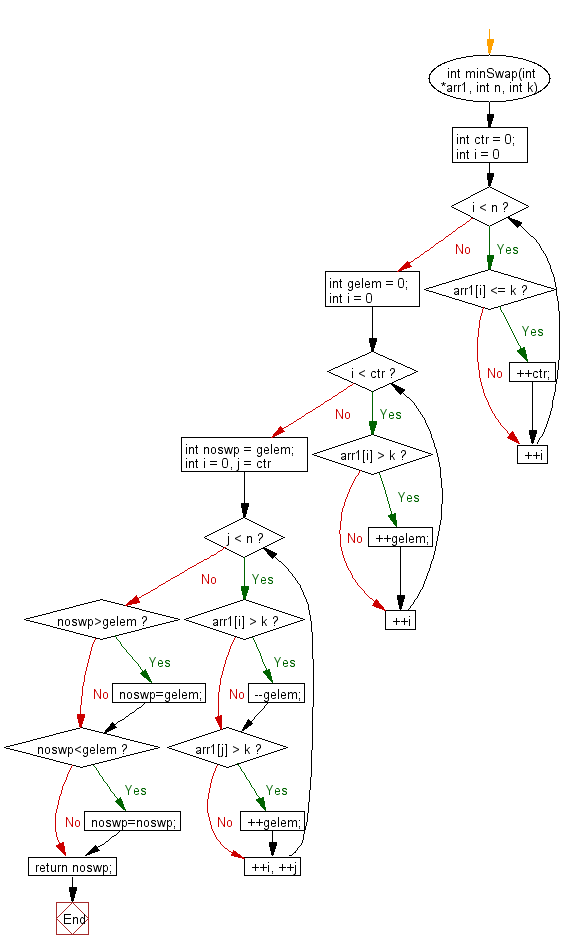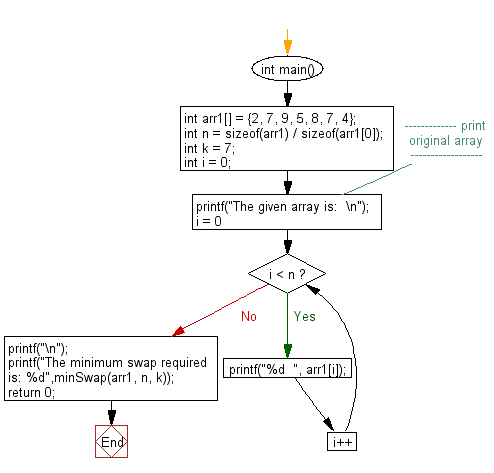﻿ C exercises: Find minimum number of swaps required to gather all elements less than or equals to k - w3resource# C Exercises: Find minimum number of swaps required to gather all elements less than or equals to k

## C Array: Exercise-105 with Solution

Write a program in C to find minimum number of swaps required to gather all elements less than or equals to k.

Sample Solution:

C Code:

``````#include<stdio.h>
int minSwap(int *arr1, int n, int k)
{
int ctr = 0;
for (int i = 0; i < n; ++i)
if (arr1[i] <= k)
++ctr;
int gelem = 0;
for (int i = 0; i < ctr; ++i)
if (arr1[i] > k)
++gelem;
int noswp = gelem;
for (int i = 0, j = ctr; j < n; ++i, ++j)
{
if (arr1[i] > k)
{
--gelem;
}
if (arr1[j] > k)
{
++gelem;
}
}
if(noswp>gelem)
{
noswp=gelem;
}
if(noswp<gelem)
{
noswp=noswp;
}
return noswp;
}
int main()
{
int arr1[] = {2, 7, 9, 5, 8, 7, 4};
int n = sizeof(arr1) / sizeof(arr1);
int k = 7;
int i = 0;
//------------- print original array ------------------
printf("The given array is:  \n");
for(i = 0; i < n; i++)
{
printf("%d  ", arr1[i]);
}
printf("\n");
//-----------------------------------------------------------
printf("The minimum swap required is: %d",minSwap(arr1, n, k));
return 0;
}
```
```

Sample Output:

```The given array is:
2  7  9  5  8  7  4
The minimum swap required is: 2
```

Pictorial Presentation:Flowchart 1:Flowchart 2:C Programming Code Editor:

Improve this sample solution and post your code through Disqus.

﻿